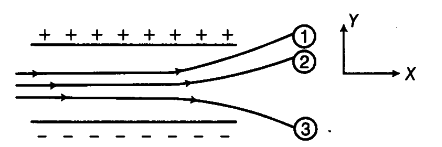# The given figure shows tracks of three charged particles in a uniform electrostatic field

The given figure shows tracks of three charged particles in a uniform electrostatic field. Give the signs of the three charges. Which particle has the highest charge to mass ratio?We know that a positively charged particle is attracted towards the negatively charged plate and a negatively charged particle is attracted towards the positively charged plate.
Here, particle 1 and particle 2 are attracted towards positive plate that means particle 1 and particle 2 are negatively charged. Particle 3 is attracted towards negatively charged plate so it is positively charged.
As the deflection in the path of a charged particle is directly proportional to the charge/mass ratio.
y ∝ q/m
Here, the deflection in particle 3 is maximum. So, the charge to mass ratio of particle 3 is maximum.